Home | Study Links | Poems |

## Planet Diameters

To begin with, a few ideas on Spirals, Circles, and the Numbers 3,6,9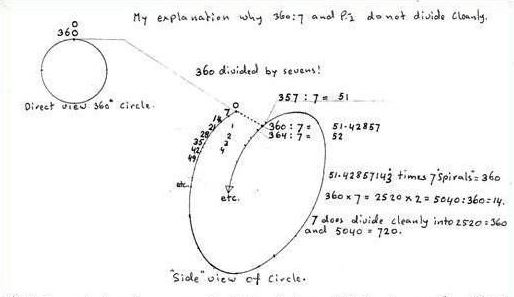Spirals to form a Torus or donut shape, 21, 42, 63, etc.
Bible verse: "That which hath been is now; and that which is to be hath already been" Ecc 3:15
The past is now; and future is already past. A circular description perhaps?

360 / 7 and .14 28 57... x 360= 51.42857142857... repeating 14 28 57. And 360 / .14 28 57...= 2520= 7 x 360

.14 28 57... x 1= .14 28 57...
.14 28 57... x 2= .28 57 14...
.14 28 57... x 3= .42 85 71...
.14 28 57... x 4= .57 14 28...
.14 28 57... x 5= .71 42 85...
.14 28 57... x 6= .85 71 42...
.14 28 57... x 7= 1
.14 28 57... x 8= 1.14 28 57...
.14 28 57... x 9= 1.28 57 14...
Etc.
From 21 on, the numbers 3, 6 and 9 are included.
.14 28 57...x 21= 3
.14 28 57...x 42= 6
.14 28 57...x 63= 9Planet Ratios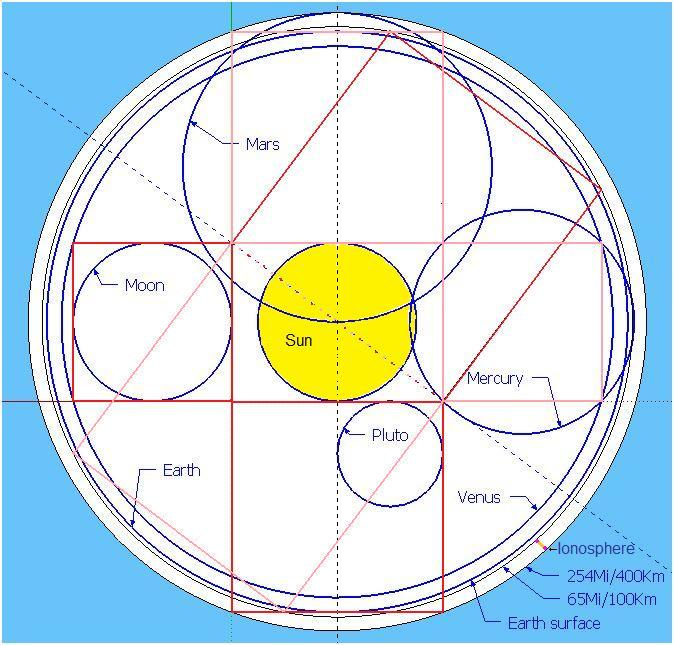Planets, perfect ratios and the Pythagorean 3-4-5 drawings where the Moon fits the square of 3, and the Earth's ratio/measurement is 11 (4+3+4). It is possible to get accurate planet diameters by using the Pythagorean drawing! Furthermore, the two circles just beyond Earth's physical circle represent our atmospheric layers. Measured here as 65 miles +/- 100 km for the first layers and the next one at 254 miles +/- 400 km. (ionosphere)

9 Planet diameters: All planet diameters divided by the ratio number = 720. For example Earth 7920 / 11= 720
1. Mercury --= 3054.70 -mi<2=>(9331200) ---= 4.24-------<2=>--------18
2. Venus ----= 7517.02--mi<2=>(56505600) --= 10.44------<2=>------109
3. Earth -----= 7920------mi<2=>(62726400) --= 11---------<2=>-------121
----Moon-----= 2160-----mi<2=>(4665600) ----= 3-----------<2=>---------9
4. Mars-------= 4213.69-mi<2=>(17755200) ---= 5.85-------<2=>--------34.25 X 4 = " 137 "
5. Jupiter----= 87120----mi<2=>(7589894400)= 121--------<2=>----14641---------(Jupiter =-------11 Earth diameters.)
6. Saturn-----= 73312.83mi<2=>(5374771200)= 101.82----<2=>----10368---------(Saturn =--------24 Mercury diameters.)
7. Uranus---- = 31680---mi<2=>(1003622400) = 44---------<2=>------1936---------(Uranus =---------4 Earth diameters.)
8. Neptune--= 30547.62mi<2=>(933120000) -= 42.42-----<2=>------1800---------(Neptune =-----10 Mercury diameters.)
9. Pluto ------= 1440-----mi<2=>(2073600) ----= 2----------<2=>----------4
Total of 9 Planet ratio/measurements ------= 342.78... Times 3.5 equals the diameter of the Sun. (Moon excluded)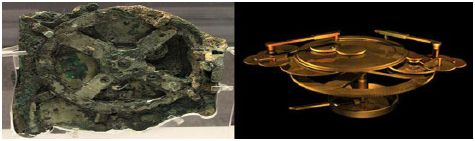A possible link between the Antikythera Mechanism and Planet size ratio's.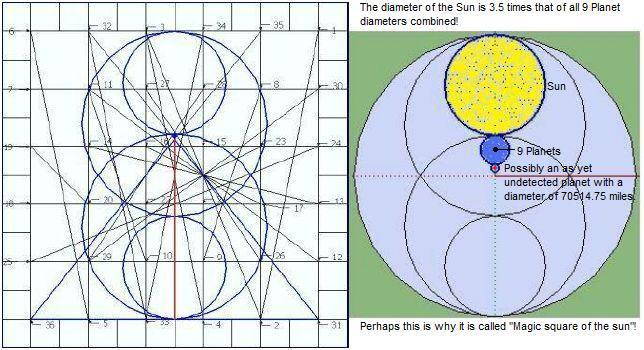Based on the magic square of the sun design and the previously established 9 Planets ratio number 342.78..., it suggests that beyond Pluto there exists another large planet with a diameter of up to 70514.75 miles! Or several smaller planets perhaps. So, 9 known planet diameters plus the possibility of several more, multiplied by 2.5, will equal the diameter of the Sun! Perhaps the asteroid belt was one of them.

Sun diameter measurements:
A Sun diam. of 863812.47…mi-2= 746172000000<27>ratio 1199.73… 2= 1439375.00 : 3.5 = 342.78...2= 117500<14>
A Sun diam. of 864000 mi----- 2= 746496000000<36>ratio 1200-------2= 1440000.00 : 3.5 = 342.857142857…
A Sun diam. of 864360 mi----- 2= 747118209600<45>ratio 1200.5-----2= 1441200.25 : 3.5 = 343(7x7x7)= 117649<28>

And a few more observations:
Sun ratio-----1200 = 864000 (diameter in miles) divided by 2000 is 432, and 4322= A lightspeed of 186624 mps
Sun ratio -1200.5 = 864360 (1200.5 sits between a prime and a non-prime number, 1201 and 1200.)
1200.5 x 2 = 2401 and interestingly 72= 49, and 492= 2401 or 7 x 7 = 49 x 7 = 343 x 7 = 2401
A Sun diameter of 864360 miles divided by the most distant planet Pluto diameter 1440 = 600.25 x 4 = 2401
864360 / 2401 = 360

Earth's atmospheric layers sandwich the ionosphere between 65 miles (+/- 100 km) and 254 miles (+/- 400 km).
65 x 2 = 130 + 7920 = 8050 miles2 = 64802500 = ratio 11.18...2 = 125
253.69...x 2 = 507.38...+ 7920= 8427.38...miles2= 71020800= ratio 11.70...2= 137= (8427.38...mi= 2 Mars widths.)Another example of accuracy concerns the two great lights in the sky and the fact that when viewed from Earth they appear to have identical diameters. The Sun is 400 times wider than the Moon (400 x 2160), and the distance between Earth and Sun is 400 times the distance between Earth and Moon, which is a 400 to 1 ratio!

God speaking to Job: "Where wast thou when I laid the foundations of the earth? declare, if thou hast understanding. 5 Who hath laid the measures thereof, if thou knowest? or who hath stretched the line upon it? 6 Whereupon are the foundations thereof fastened? or who laid the corner stone thereof; 7 When the morning stars sang together, and all the sons of God shouted for joy?"Job 38: 4 Kjv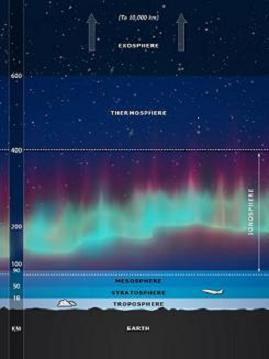The ionosphere (layer between 100 and 400 km) is the area where the Northern and Southern Auroras occur, and as the planet ratio drawing clearly shows, it all shows perfect design like a clock or a precision timepiece!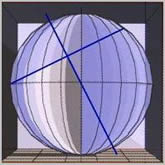The 3° difference between this Axial tilt method @ 26.565° and Earth's present-day tilt @ 23.5°
The arms of this cross multiplied by 1.25 equals it's length."In the beginning God created the heaven and the earth." (Genesis 1:1)
The gematria ( sum, using numerical values of its letters ) of these first 7 words of the book of Genesis is 2701

"In the beginning was the Word and the Word was with God, and the Word was God. 2 The same was in the beginning with God. 3 All things were made by him; and without him was not anything made that was made...14 And the Word was made flesh, and dwelt among us," (John 1:1)

"Who hath measured the waters in the hollow of his hand, and meted out heaven with the span, and comprehended the dust of the earth in a measure, and weighed the mountains in scales, and the hills in a balance?" (Isaiah 40:12)

Click on the image for its origin.Continue with Stonehenge